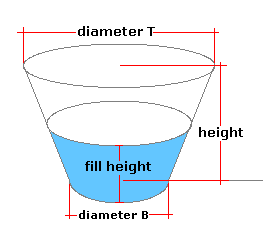Spike's Calculators

# Partial Volume in a Truncated Funnel Tank/Container - MetresCalculate the amount of liquid in a partially filled truncated funnel tank or container.

This calculator uses metres for measurements.

##### Approximate weight of Fuel or Water
```one litre of water = 1 kilogram
one litre of diesel fuel = 0.832 kilogram
one litre of gas = 0.75 kilogram
```

### Truncated Funnel

Top Diameter m
Bottom Diameter m
Funnel Height m
Depth of Liquid m
Liquid Weight-Kilograms per Litre kg/L

#### Results:

###### Tank Volume and Capacity
Cubic Metres
Cubic Inches in³
Cubic Feet ft³
Capacity in Litres L
Capacity in Hectolitres hl
Capacity in US Gallons gal US
###### Amount of Liquid Inside Tank
In Cubic Metres
In Cubic Feet ft³
In Litres L
In Hectolitres hl
In US Gallons gal US
In Imperial Gallons gal Imperial
###### Weight of Liquid and Fill Amount
Weight of Liquid kg
To Fill this Tank, Add Litres

#### Calculation

1. enter the top diameter of the truncated funnel in metres
2. the bottom diameter in metres
3. the height of the funnel in metres
4. the depth of the liquid inside the tank in metres
5. the weight of the liquid in kilograms per litre

#### Results

1. the volume of the tank in cubic metres
2. the volume in cubic inches
3. the volume in cubic feet
4. the total amount of liquid the tank can hold in litres
5. the tank's capacity in hectolitres
6. the tank's capacity in US gallons
7. the amount of liquid inside the tank in cubic metres
8. the amount of liquid inside the tank in cubic feet
9. the amount of liquid inside the tank in litres
10. the amount of liquid inside the tank in hectolitres
11. the amount of liquid inside the tank in US gallons
12. the amount of liquid inside the tank in Imperial Gallons
13. the weight of the liquid inside the tank based on kilograms per litre
14. the amount of liquid it will take to top up this tank in litres

##### Formula
```V = d*π/3*p²+pa+a²
where V is the volume of the tank
d the fill height of the liquid
Π = 3.14159265
p diameter @ fill height
a half the tanks'  bottom diameter
```
##### Conversions
```one cubic metre (m³) = 10 hectolitres (hl)
one cubic metre (m³) = 1000 litres (L)
one cubic metre (m³) = 61023.7440947323 cubic inches (in³)
one cubic metre (m³) =  35.3146667214886 cubic feet (ft³)
one cubic metre (m³) = 264.172052358148 gallons (gal) US
one cubic metre (m³) = 219.969248299088 gallons (gal) Imperial
```

### Funnel Tank/Container (Truncated)#### Filled